# Common Core: 1st Grade Math : Add a Two-Digit Number to a One-Digit Number, and Two-Digit Number and a Multiple of 10: CCSS.MATH.CONTENT.1.NBT.C.4

## Example Questions

← Previous 1

### Example Question #1 : Add A Two Digit Number To A One Digit Number, And Two Digit Number And A Multiple Of 10: Ccss.Math.Content.1.Nbt.C.4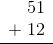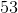Explanation:

When we add two digit numbers, we start by adding the numbers in the ones place.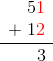Next, we need to add the numbers in the tens place.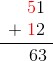The final answer is### Example Question #1 : Add A Two Digit Number To A One Digit Number, And Two Digit Number And A Multiple Of 10: Ccss.Math.Content.1.Nbt.C.4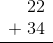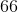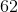Explanation:

When we add two digit numbers, we start by adding the numbers in the ones place.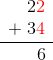Next, we need to add the numbers in the tens place.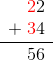The final answer is### Example Question #1 : Add A Two Digit Number To A One Digit Number, And Two Digit Number And A Multiple Of 10: Ccss.Math.Content.1.Nbt.C.4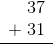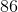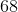Explanation:

When we add two digit numbers, we start by adding the numbers in the ones place.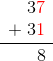Next, we need to add the numbers in the tens place.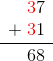The final answer is### Example Question #4 : Place Value And Properties Of Operations To Add And Subtract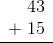Explanation:

When we add two digit numbers, we start by adding the numbers in the ones place.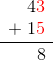Next, we need to add the numbers in the tens place.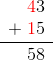The final answer is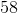### Example Question #1 : Place Value And Properties Of Operations To Add And Subtract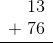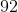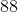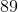Explanation:

When we add two digit numbers, we start by adding the numbers in the ones place.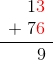Next, we need to add the numbers in the tens place.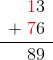The final answer is### Example Question #1 : Add A Two Digit Number To A One Digit Number, And Two Digit Number And A Multiple Of 10: Ccss.Math.Content.1.Nbt.C.4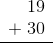Explanation:

When we add two digit numbers, we start by adding the numbers in the ones place.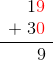Next, we need to add the numbers in the tens place.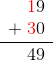The final answer is### Example Question #7 : Place Value And Properties Of Operations To Add And Subtract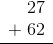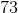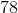Explanation:

When we add two digit numbers, we start by adding the numbers in the ones place.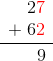Next, we need to add the numbers in the tens place.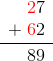The final answer is### Example Question #8 : Place Value And Properties Of Operations To Add And Subtract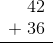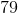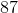Explanation:

When we add two digit numbers, we start by adding the numbers in the ones place.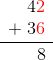Next, we need to add the numbers in the tens place.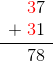The final answer is### Example Question #2 : Add A Two Digit Number To A One Digit Number, And Two Digit Number And A Multiple Of 10: Ccss.Math.Content.1.Nbt.C.4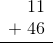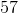Explanation:

When we add two digit numbers, we start by adding the numbers in the ones place.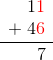Next, we need to add the numbers in the tens place.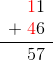The final answer is### Example Question #11 : Add A Two Digit Number To A One Digit Number, And Two Digit Number And A Multiple Of 10: Ccss.Math.Content.1.Nbt.C.4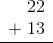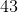Explanation:

When we add two digit numbers, we start by adding the numbers in the ones place.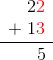Next, we need to add the numbers in the tens place.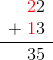The final answer is← Previous 1

### All Common Core: 1st Grade Math Resources